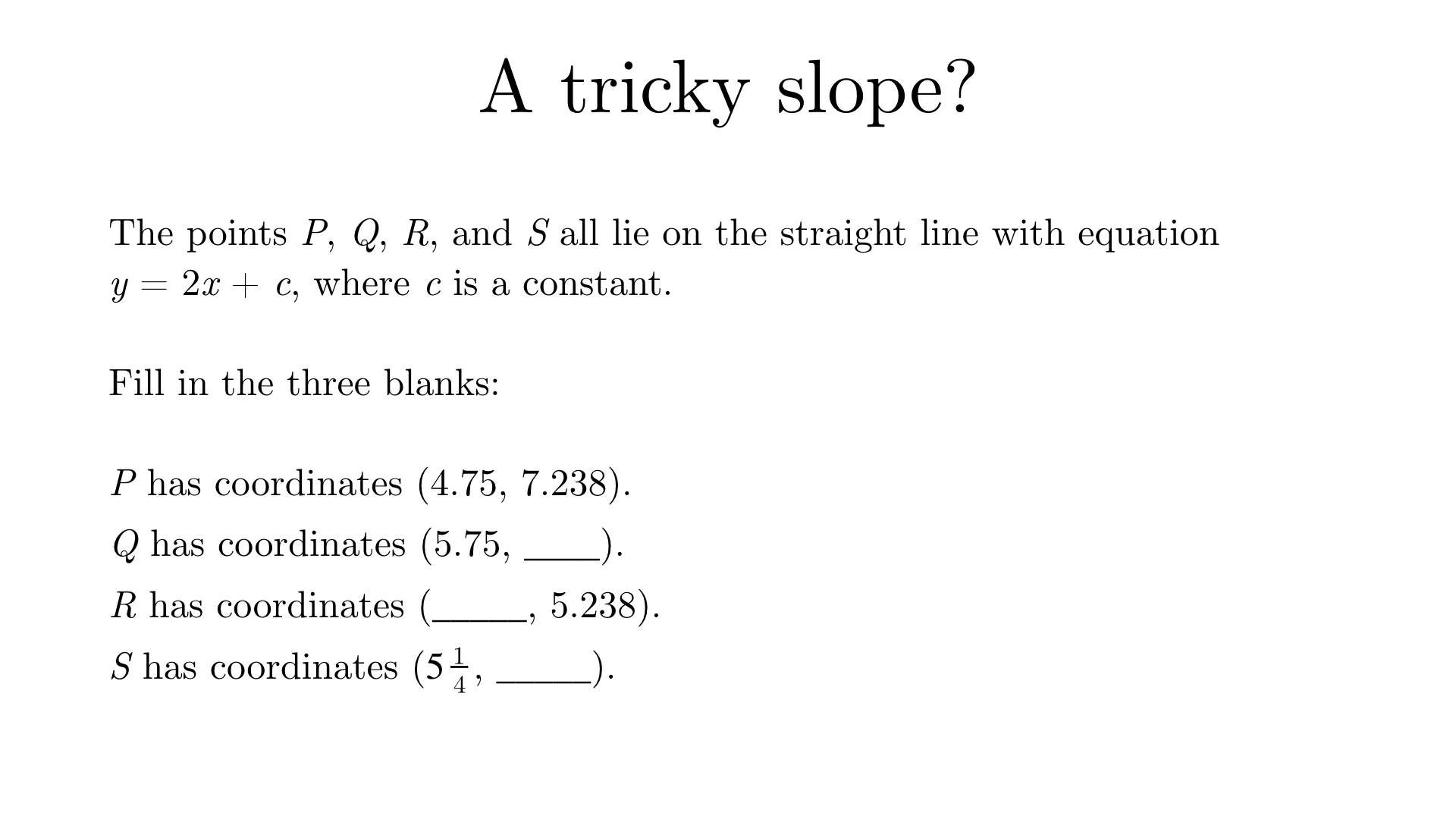Coordinates of Q
On a straight line, a gradient of 2 means that for every step to the right, you go 2 steps up. Therefore, Q has coordinates $$(5.75, 9.238)$$. There is no need to work out the intercept, $$c$$.
Coordinates of R
R has coordinates $$(3.75, 5.238)$$.
Coordinates of S
S has coordinates $$(5.25, 8.238)$$.